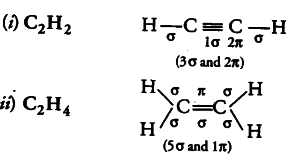# What is the total number of sigma and pi bonds

What is the total number of sigma and pi bonds in the following molecules?
(i) \$C_{2}H_{2}\$ (ii) \$C_{2}H_{4}\$